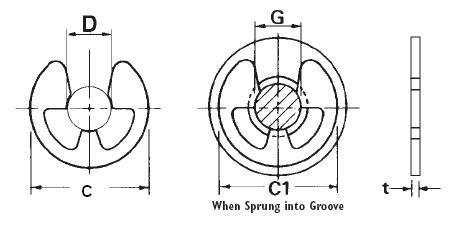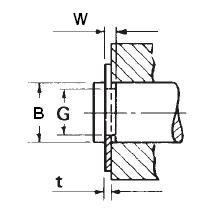### ETW-009

Display Units: inches |  metric
Request Quick Quote for this part.
 Ring Specs (D) Free Diameter: 0.354 +0.000 / -0.006 in. (t) Thickness: 0.031 +0.002 / -0.002 in. (b) Radial Wall: Groove Specs: (B) Application Diameter: 0.472 in. (G) Groove Diameter: 0.358 +0.004 / -0.000 in. (W) Groove Width: 0.035 +0.004 / -0.000 in. Groove Depth: 0.057 in. Other Specs Approximate Weight per 1000: (C) Free Outside Diameter: 0.709 in. (Pg) Theoretical Thrust Load Capacity - Groove Yield:Notes: Yield Strength of Groove Material (Ys): 45,000 psi. Calculated using a safety factor (K) of 2Equation:Pg = [ Cf * B * d * Ys * pi ] / K    = [ (0.333) * (0.472 in.) * (0.057 in.) * (45,000 psi) * 3.14 ] / 2 632 lbs. (Pr) Theoretical Thrust Load Capacity - Ring Shear:Notes: Ring Material: Carbon Spring Steel (SAE 1060-1090). Shear Strength of Ring Material* (Ss): 120,000 psi. Calculated using a safety factor (K) of 3Equation:Pr = [ Cf * B * t * Ss * pi ] / K    = [ (0.333) * (0.472 in.) * (0.031 in.) * (120,000 psi) * 3.14 ] / 3*Shear Strength of Material value is for Carbon Spring Steel material only. 611 lbs.